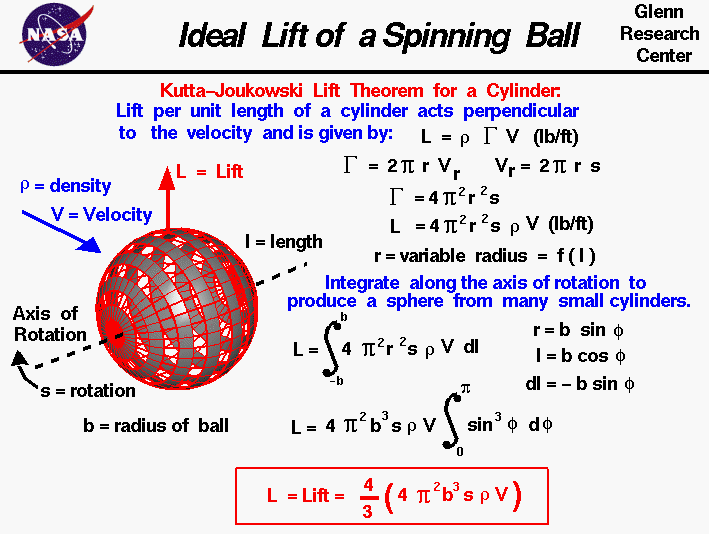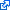+ Text Only Site
+ Non-Flash Version
+ Contact GlennAll that is necessary to create lift is to turn a flow of air. The airfoil of a wing turns a flow, and so does a rotating cylinder. A spinning ball also turns a flow and generates an aerodynamic lift force. The details of how a spinning ball creates lift are fairly complex. Next to any surface, the molecules of the air stick to the surface, as discussed in the properties of air slide. This thin layer of molecules entrains or pulls the surrounding flow of air. For a spinning ball the external flow is pulled in the direction of the spin. If the ball is not translating, we have a spinning, vortex-like flow set up around the spinning ball, neglecting three-dimensional and viscous effects in the outer flow. If the ball is translating through the air at some velocity, then on one side of the ball the entrained flow opposes the free stream flow, while on the other side of the ball, the entrained and free stream flows are in the same direction. Adding the components of velocity for the entrained flow to the free stream flow, on one side of the ball the net velocity is less than free stream; while on the other, the net velocity is greater than free stream. The flow is then turned by the spinning ball, and a force is generated. Because of the change to the velocity field, the pressure field is also altered around the ball. The magnitude of the force can be computed by integrating the surface pressure times the area around the ball. The direction of the force is perpendicular (at a right angle) to the flow direction and perpendicular to the axis of rotation of the ball. On the figure at the left, we show the geometry of the spinning ball. A ball of radius b rotates at speed s measured in revolutions per second. A black dashed line indicates the axis of rotation of the ball, and the ball rotates clock-wise, when viewed along the axis from the lower left. The ball has been sliced into a large number of grey-colored sections along the axis of rotation. The air with velocity V and density rho strikes the ball from the upper left. The resulting lift force L is perpendicular to the air velocity and the axis of rotation. To determine the ideal lift force on the ball, we consider the spinning ball to be composed of an infinite number of very small, grey-colored, rotating cylinders. Adding up (integrating) the lift of all of the cylinders along the axis gives the ideal lift of the ball. The Kutta-Joukowski lift theorem for a single cylinder states the lift per unit length L is equal to the density rho of the air times the strength of the rotation Gamma times the velocity V of the air: L = rho * Gamma * V The strength of rotation is directly related to the rotational speed of the cylinder. For a cylinder of radius r rotating at angular speed s, the surface of the cylinder moves at speed Vr given by: Vr = 2 * pi * r * s Then the strength of rotation is equal to: Gamma = 2 * pi * r * Vr Combining these equations: Gamma = 4 * pi^2 * r^2 * s And substituting this value into the Kutta-Joukowski lift equation give: L = 4 * pi^2 * r^2 * s * rho * V This is the lift per unit length for a single small cylinder and is measured in force per length (lbs/ft). The ball is composed of an infinite number of cylinders with the radius of the cylinders changing along the axis of rotation. r = f (l) where l is a length measured along the axis of rotation. For a ball of radius b we have to integrate the lift per unit length along the axis of rotation from -b to +b. If we let S be the symbol for integration and dl be an increment of l: L = S (4 * pi^2 * r^2 * s * rho * V) dl If we let phi be an angle from the center of the ball along the axis of rotation: r = b sin(phi) l = b cos(phi) dl = -b sin(phi) d(phi) Then: L = 4 * pi^2 * b^3 * s * rho * V * S (sin (phi))^3 d(phi) with the limits of integration going from zero to pi. Performing the integration: L = (4 * pi^2 * b^3 * s * rho * V) * 4 / 3 Let's investigate the lift on a spinning ball by using a Java simulator. Due to IT security concerns, many users are currently experiencing problems running NASA Glenn educational applets. The applets are slowly being updated, but it is a lengthy process. If you are familiar with Java Runtime Environments (JRE), you may want to try downloading the applet and running it on an Integrated Development Environment (IDE) such as Netbeans or Eclipse. The following are tutorials for running Java applets on either IDE: NetbeansEclipseThis page shows an interactive Java applet with flow past a spinning ball. There is a plotter and the calculated lift is displayed. The left window shows a view of a ball placed in a flow of air. The ball is a foot in diameter and it is moving 100 miles an hour. You can spin the ball by using the slider below the view window or by backspacing over the input box, typing in your new value and hitting the Enter key on the keyboard. On the right is a graph of the lift versus spin. The red dot shows your conditions. Below the graph is the numerical value of the lift. You can display either the lift value (in English or Metric units) or the lift coefficient by using the choice buttons surrounding the output box. Click on the choice button and select from the drop-menu. As an experiment, set the spin to 200 rpm (revolutions per minute) and note the amount of lift. Now increase the spin to 400 rpm. Did the lift increase or decrease? Set the spin to -400 rpm. What is the value of lift? Which way would this ball move? You can download a copy of this program by clicking on this button: You should save the file "FoilB.zip" to your computer, then "Extract" the files. Click on "Foil.html" to launch the program. Have fun! You can further investigate the lift of a spinning ball, and a variety of other shapes by using the FoilSim III Java Applet. You can also download your own copy of FoilSim to play with for free. There is also a Java Applet called CurveBall to help you explore the aerodynamics of big league pitching. It computes the path of a thrown curveball. And there is a new (Aug, 2010) Java applet called SoccerNASA that models the aerodynamics of a soccer ball. A kicked soccer ball curves in flight because of aerodynamic forces. Be particularly aware of the simplifying assumptions that have gone into this analysis. The type of flow field shown on this figure is called an ideal flow field. We have produced the ideal flow field by superimposing the flow field from an ideal vortex centered on the ball with a uniform free stream flow. There is no viscosity in this model, and therefore, no boundary layer on the ball, even though viscosity is the real origin of the circulating flow! The equation given above describes the ideal lift force generated on a smooth, rotating ball. We can use this description as a first order, or preliminary, estimate of the lift on a baseball or a soccer ball. But in reality, the flow around a spinning baseball or soccer ball is very complex. Viscosity generates a boundary layer on the ball and the stitches used to hold the covering of the ball together stick up out of the boundary layer and disturb both the boundary layer and free stream flow. On the ball, the boundary layer transitions to turbulent flow which affects the amount of aerodynamic drag. The stitches are not symmetrically distributed around the ball. So the real flow around the ball is separated, unsteady, and not uniform. To account for these real-world effects that we have neglected in our ideal flow model, we define a lift coefficient. The lift coefficient is an experimentally determined factor that is multiplied times the ideal lift value to produce a real lift value. The ideal simplified model gives the first order effects and tells us the relative importance of the factors that affect the lift force on the ball, while all of the complex factors are modeled by the lift coefficient. Activities:Guided ToursObjects that Generate Lift:CurveBall - Baseball Simulation:Navigation ..NASA Baseball Home Page NASA Soccer Home Page Beginner's Guide Home Page+ Inspector General Hotline + Equal Employment Opportunity Data Posted Pursuant to the No Fear Act + Budgets, Strategic Plans and Accountability Reports + Freedom of Information Act + The President's Management Agenda + NASA Privacy Statement, Disclaimer, and Accessibility CertificationEditor: Nancy Hall NASA Official: Nancy Hall Last Updated: Jul 28 2008 + Contact Glenn